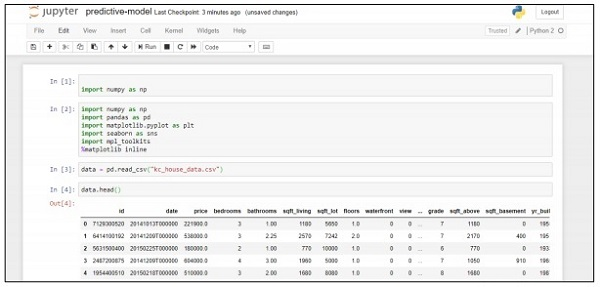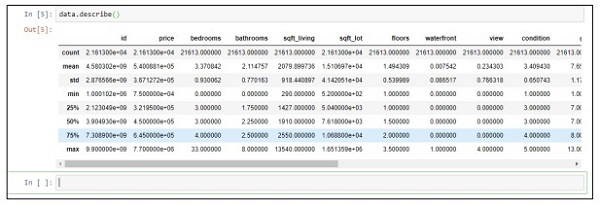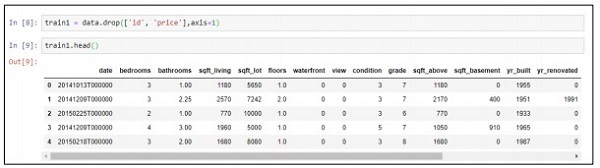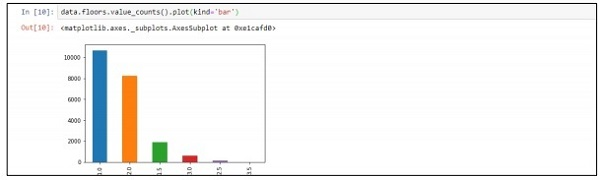# Deploying a predictive system

In this example, we will learn how to create and deploy predictive model which helps in the prediction of house prices using python script. The important framework used for deployment of predictive system includes Anaconda and “Jupyter Notebook”.

Follow these steps to deploy a predictive system −

Step 1 − Implement the following code to convert values from csv files to associated values.

```import numpy as np
import pandas as pd
import matplotlib.pyplot as plt
import seaborn as sns
import mpl_toolkits

%matplotlib inline
```

The above code generates the following output −Step 2 − Execute the describe function to get the data types included in attributed of csv files.

```data.describe()
```Step 3 − We can drop the associated values based on the deployment of the predictive model that we created.

```train1 = data.drop(['id', 'price'],axis=1)
```Step 4 − You can visualize the data as per the records. The data can be used for data science analysis and output of white papers.

```data.floors.value_counts().plot(kind='bar')
```